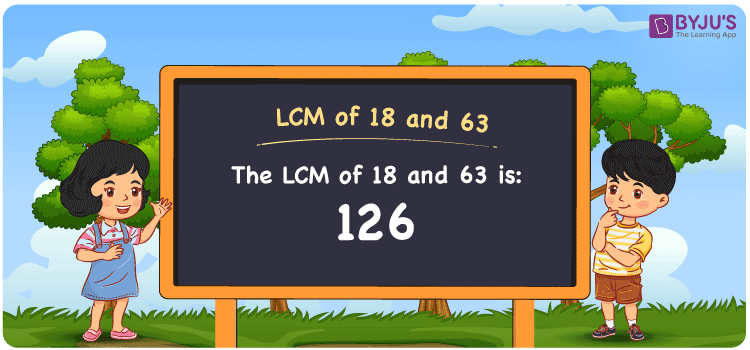# LCM of 18 and 63

LCM of 18 and 63 is 126. LCM, which is Least Common Multiple or Lowest Common Multiple, is the smallest positive integer that is divisible by the given set of numbers. Consider the example for finding the LCM of 18 and 63. The answer is 126. 126 is divisible by both 18 and 63. Even 252 is divisible by 18 and 63, however it is not the LCM for 18 and 63. The smaller number than 252 is 126 which is divisible by both 18 and 63. Hence 126 is the Least Common Multiple for 18 and 63. You can use the Prime Factorization and Division method to find the LCM of any 2 numbers.

## What is LCM of 18 and 63

The Least Common Multiple or Lowest Common Multiple of 18 and 63 is 126.## How to Find LCM of 18 and 63?

LCM of 18 and 63 can be determined using three methods:

• Prime Factorisation
• Division method
• Listing the multiples

### LCM of 18 and 63 Using Prime Factorisation Method

In the Prime Factorisation method, the numbers 18 and 63 can be expressed as;

18 = 2 × 3 × 3

63 = 3 × 3 × 7

The LCM is obtained by common and uncommon factors of 18 and 63.

LCM (18, 63) = 2 × 3 × 3 × 7 = 126

### LCM of 18 and 63 Using Division Method

In the Division Method, the numbers 18 and 63 are divided together by common prime divisors. The product of all the prime divisors, of 18 and 63 forms the LCM.

 2 18 63 3 9 21 3 3 7 7 1 7 × 1 1

LCM (18, 63) = 2 × 3 × 3 × 7 = 126

### LCM of 18 and 63 Using Listing the Multiples

By listing all the multiples of 18 and 63, we can identify the LCM. Below is the list of multiples for 18 and 63

Multiples of 18:

18, 36, 54, 72, 90, 108, 126, 144, 162, 180, 198…

Multiples of 63:

63, 126, 189, 252…

LCM (18, 63) = 126

## Video Lesson on Applications of LCM## Solved Examples

What is the smallest number that is divisible by both 18 and 63?

Answer: 126 is the smallest number that is divisible by both 18 and 63.

What is the LCM for 3, 9, 18 and 63?

Answer: LCM for 3, 9, 18 and 63 is 126 as 3 and 9 are also the factors of 18 and 63.

## Frequently Asked Questions on LCM of 18 and 63

### What is the LCM of 18 and 63?

The LCM of 18 and 63 is 126.

### Is the LCM of 18 and 63 same as the HCF of 18 and 63?

No. Least Common Multiple of 18 and 63 is 126 and the Highest Common Factor of 18 and 63 is 9.

### Is the LCM and LCD both the same for 18 and 63?

Yes. The Least Common Multiple and Lowest Common Divisor are both the same for 18 and 63, which is 126.

### What are the first 3 common multiples of 18 and 63?

The first three common multiples of 18 and 63 are 126, 252, and 378.

### Is the LCM of 9 and 18 the same as the LCM of 9 and 63?

No. LCM of 9 and 18 is 18, whereas the LCM of 9 and 63 is 63. They are not the same.